Courses

# Critical Thickness of Insulation & Heat Transfer From Extended Surfaces (Fins) - MCQ Test 2

## 30 Questions MCQ Test RRB JE for Mechanical Engineering | Critical Thickness of Insulation & Heat Transfer From Extended Surfaces (Fins) - MCQ Test 2

Description
This mock test of Critical Thickness of Insulation & Heat Transfer From Extended Surfaces (Fins) - MCQ Test 2 for Mechanical Engineering helps you for every Mechanical Engineering entrance exam. This contains 30 Multiple Choice Questions for Mechanical Engineering Critical Thickness of Insulation & Heat Transfer From Extended Surfaces (Fins) - MCQ Test 2 (mcq) to study with solutions a complete question bank. The solved questions answers in this Critical Thickness of Insulation & Heat Transfer From Extended Surfaces (Fins) - MCQ Test 2 quiz give you a good mix of easy questions and tough questions. Mechanical Engineering students definitely take this Critical Thickness of Insulation & Heat Transfer From Extended Surfaces (Fins) - MCQ Test 2 exercise for a better result in the exam. You can find other Critical Thickness of Insulation & Heat Transfer From Extended Surfaces (Fins) - MCQ Test 2 extra questions, long questions & short questions for Mechanical Engineering on EduRev as well by searching above.
QUESTION: 1

### It is proposed to coat a 1 mm diameter wire with enamel paint (k = 0.1W/mK) to increase heat transfer with air. If the air side heat transfer coefficient is 100 W/m2K, then optimum thickness of enamel paint should be:

Solution:

Ans. (b) Critical radius of insulation (rc) =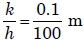= 1mm

∴ Critical thickness of enamel point =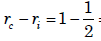= 0.5 mm

QUESTION: 2

Solution:

Ans. (c)

QUESTION: 3

### Upto the critical radius of insulation

Solution:

Ans. (c) The thickness upto which heat flow increases and after which heat flow decreases is termed as Critical thickness. In case of cylinders and spheres it is called 'Critical radius'.

QUESTION: 4

A hollow pipe of 1 cm outer diameter is to be insulated by thick cylindrical insulation having thermal conductivity 1 W/mK. The surfaceheat transfer coefficient on the insulation surface is 5 W/m2K. What isthe minimum effective thickness of insulation for causing the reduction in heat leakage from the insulated pipe?

Solution:

Ans. (c) Critical radius of insulation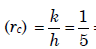= 0.2m = 20cm
∴ Critical thickness of insulation ( Δr)C = rc − r1 = 20 - 0.5 = 19.5cm

QUESTION: 5

A copper wire of radius 0.5 mm is insulated with a sheathing ofthickness 1 mm having a thermal conductivity of 0.5 W/m – K. Theoutside surface convective heat transfer coefficient is 10 W/m2 – K. Ifthe thickness of insulation sheathing is raised by 10 mm, then theelectrical current-carrying capacity of the wire will:

Solution:

Ans. (a)

QUESTION: 6

A copper pipe carrying refrigerant at – 200 C is covered by cylindrical insulation of thermal conductivity 0.5 W/m K. The surface heat transfer coefficient over the insulation is 50 W/m2 K. The critical thickness ofthe insulation would be:

Solution:

Ans. (a) Critical radius of insulation ( rc) =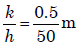= 0.5m = 0.01m

QUESTION: 7

The temperature variation in a largeplate, as shown in the given figure,would correspond to which of thefollowing condition (s)?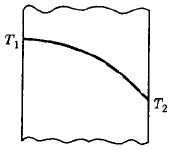1. Unsteady heat
2. Steady-state with variation of k
3. Steady-state with heat generation
Select the correct answer using the codes given below:
Codes:

Solution:

Ans. (a)

QUESTION: 8

Water jacketed copper rod “D” m in diameter is used to carry the current. The water, which flows continuously maintains the rod temperature at Toi C during normal operation at “I” amps. The electrical resistance of the rod is known to be “R” Ω /m. If the coolant water ceased to be available and the heat removal diminished greatly, the rod would eventually melt. What is the time required for melting to occur if the melting point of the rod material is Tmp?
[Cp = specific heat, ρ = density of the rod material and L is the length of the rod]

Solution:

Ans. (a)

QUESTION: 9

Consider the following statements:
1. Under certain conditions, an increase in thickness of insulation may increase the heat loss from a heated pipe.
2. The heat loss from an insulated pipe reaches a maximum when theoutside radius of insulation is equal to the ratio of thermalconductivity to the surface coefficient.
3. Small diameter tubes are invariably insulated.
4. Economic insulation is based on minimum heat loss from pipe.

Of these statements

Solution:

Ans. (c)

QUESTION: 10

Consider the following statements:

1. Under certain conditions, an increase in thickness of insulation mayincrease the heat loss from a heated pipe.

2. The heat loss from an insulated pipe reaches a maximum when theoutside radius of insulation is equal to the ratio of thermalconductivity to the surface coefficient.

3. Small diameter tubes are invariably insulated.

4. Economic insulation is based on minimum heat loss from pipe.Of these statements

Solution:

Ans. (c)

QUESTION: 11

An electric cable of aluminium conductor (k = 240 W/mK) is to be insulated with rubber (k = 0.15 W/mK). The cable is to be located in air (h = 6W/m2). The critical thickness of insulation will be:

Solution:

Ans. (c)

QUESTION: 12

Match List-I (Parameter) with List-II (Definition) and select the correct answer using the codes given below the lists: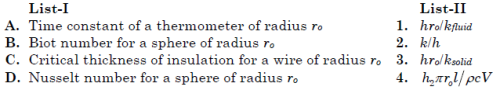Nomenclature: h: Film heat transfer coefficient, ksolid: Thermal conductivity of solid, kfluid: Thermal conductivity of fluid, ρ: Density,
c: Specific heat, V: Volume, l: Length.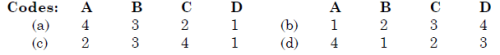Solution:

Ans. (a)

QUESTION: 13

What is the critical radius of insulation for a sphere equal to?k = thermal conductivity in W/m-K
h = heat transfer coefficient in W/m2K

Solution:

Ans. (b) Critical radius of insulation for sphere in 2k/h and for cylinder is k/h

QUESTION: 14

In current carrying conductors, if the radius of the conductor is lessthan the critical radius, then addition of electrical insulation isdesirable, as

Solution:

Ans. (b)

QUESTION: 15

Provision of fins on a given heat transfer surface will be more it thereare:

Solution:

Ans. (c)

QUESTION: 16

In current carrying conductors, if the radius of the conductor is lessthan the critical radius, then addition of electrical insulation is desirable, as

Solution:

Ans. (b)

QUESTION: 17

Which one of the following is correct?
The effectiveness of a fin will be maximum in an environment with

Solution:

Ans. (a) The effectiveness of a fin can also be characterized as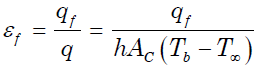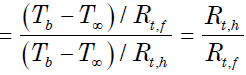It is a ratio of the thermal resistance due to convection to the thermal resistance
of a fin. In order to enhance heat transfer, the fin's resistance should be lower
than that of the resistance due only to convection.

QUESTION: 18

On heat transfer surface, fins are provided

Solution:

Ans. (c) By the use of a fin, surface area is increased due to which heat flow rate
increases. Increase in surface area decreases the surface convection resistance,
whereas the conduction resistance increases. The decrease in convection
resistance must be greater than the increase in conduction resistance in order to
increase the rate of heat transfer from the surface. In practical applications of
fins the surface resistance must be the controlling factor (the addition of fins
might decrease the heat transfer rate under some situations).

QUESTION: 19

In order to achieve maximum heat dissipation, the fin should be designed in such a way that:

Solution:

Ans. (a)

QUESTION: 20

Consider the following statements pertaining to large heat transferrate using fins:
1. Fins should be used on the side where heat transfer coefficient is small
2. Long and thick fins should be used
3. Short and thin fins should be used
4. Thermal conductivity of fin material should be large
Which of the above statements are correct?

Solution:

Ans. (d)

QUESTION: 21

Assertion (A): Nusselt number is always greater than unity.

Reason (R): Nusselt number is the ratio of two thermal resistances, onethe thermal resistance which would be offered by the fluid, if it wasstationary and the other, the thermal resistance associated with convective heat transfer coefficient at the surface.

Solution:

Ans. (a)

QUESTION: 22

Assertion (A): In a liquid-to-gas heat exchanger fins are provided in thegas side.
Reason (R): The gas offers less thermal resistance than liquid

Solution:

Ans. (c)

QUESTION: 23

Extended surfaces are used to increase the rate of heat transfer. When the convective heat transfer coefficient h = mk, the addition ofextended surface will:

Solution:

Ans. (c)

QUESTION: 24

Consider the following statements pertaining to heat transfer throughfins:
1. Fins are equally effective irrespective of whether they are on thehot side or cold side of the fluid.
2. The temperature along the fin is variable and hence the rate of heattransfer varies along the elements of the fin.
3. The fins may be made of materials that have a higher thermalconductivity than the material of the wall.
4. Fins must be arranged at right angles to the direction of flow of theworking fluid.Of these statements:

Solution:

Ans. (d)

QUESTION: 25

Addition of fin to the surface increases the heat transfer if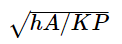is:

Solution:

Ans. (c) Addition of fin to the surface increases the heat transfer if<< 1

QUESTION: 26

A fin of length 'l' protrudes from a surface held at temperature togreater than the ambient temperature ta. The heat dissipation from thefree end' of the fin is assumed to be negligible. The temperaturegradient at the fin tip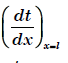is:

Solution:

Ans. (a)

QUESTION: 27

The insulated tip temperature of a rectangular longitudinal fin having an excess (over ambient) root temperature of θo is:

Solution:

Ans. (d)

QUESTION: 28

A metallic rod of uniform diameter and length L connects two heat sources each at 500°C. The atmospheric temperature is 30°C. The temperature gradient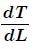at the centre of the bar will be: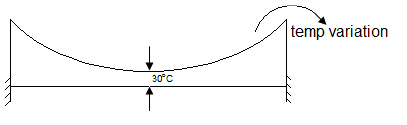Solution:

Ans. (d)

QUESTION: 29

The efficiency of a pin fin with insulated tip is:

Solution:

Ans. (b)

QUESTION: 30

A fin of length l protrudes from a surface held at temperature To; it being higher than the ambient temperature Ta. The heat dissipationfrom the free end of the fin is stated to be negligibly small, What is the temperature gradien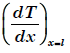at the tip of the fin?

Solution:

Ans. (a)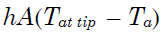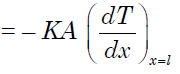= Negligibly small.

Therefore, the temperature gradientat the tip will be negligibly small
i.e. zero.